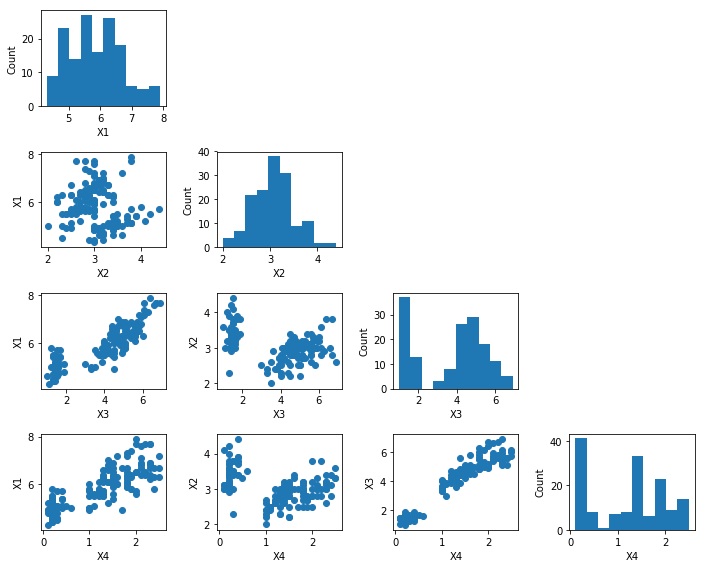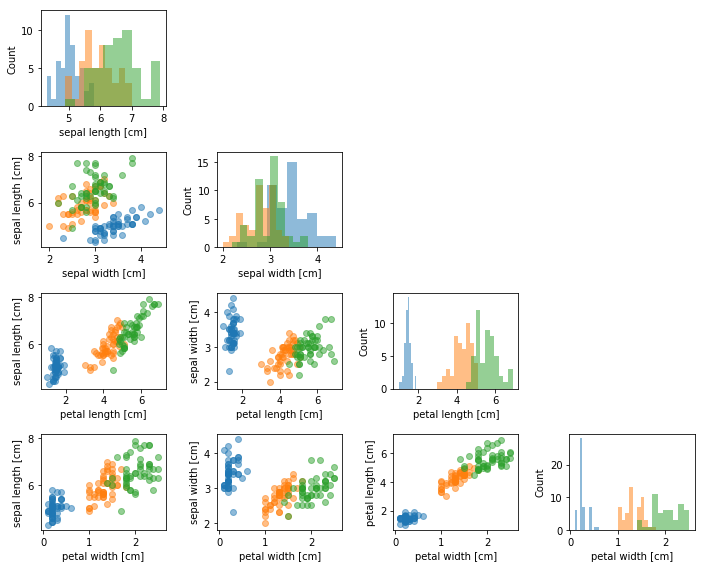# scatterplotmatrix: visualize datasets via a scatter plot matrix

A function for creating as scatter plot matrix in matplotlib

from mlxtend.plotting import scatterplotmatrix

## Overview

A matplotlib convenience function for creating a scatterplot matrix.

• -

## Example 1 - Simple Scatter Plot Matrix

import matplotlib.pyplot as plt
from mlxtend.data import iris_data
from mlxtend.plotting import scatterplotmatrix

X, y = iris_data()
scatterplotmatrix(X, figsize=(10, 8))
plt.tight_layout()
plt.show()## Example 2 - Scatter Plot Matrix with Multiple Categories

names = ['sepal length [cm]', 'sepal width [cm]',
'petal length [cm]', 'petal width [cm]']

fig, axes = scatterplotmatrix(X[y==0], figsize=(10, 8), alpha=0.5)
fig, axes = scatterplotmatrix(X[y==1], fig_axes=(fig, axes), alpha=0.5)
fig, axes = scatterplotmatrix(X[y==2], fig_axes=(fig, axes), alpha=0.5, names=names)

plt.tight_layout()
plt.show()## API

scatterplotmatrix(X, fig_axes=None, names=None, figsize=(8, 8), alpha=1.0, kwargs)

Lower triangular of a scatterplot matrix

Parameters

• X : array-like, shape={num_examples, num_features}

Design matrix containing data instances (examples) with multiple exploratory variables (features).

• fix_axes : tuple (default: None)

A (fig, axes) tuple, where fig is an figure object and axes is an axes object created via matplotlib, for example, by calling the pyplot subplot function fig, axes = plt.subplots(...)

• names : list (default: None)

A list of string names, which should have the same number of elements as there are features (columns) in X.

• figsize : tuple (default: (8, 8))

Height and width of the subplot grid. Ignored if fig_axes is not None.

• alpha : float (default: 1.0)

Transparency for both the scatter plots and the histograms along the diagonal.

• **kwargs : kwargs

Keyword arguments for the scatterplots.

Returns

• fix_axes : tuple

A (fig, axes) tuple, where fig is an figure object and axes is an axes object created via matplotlib, for example, by calling the pyplot subplot function fig, axes = plt.subplots(...)